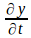## Dispersion Relations

A particle does not occupy a fixed, definite position in space. It is smeared out over a definite none zero volume in the form of a wavepacket.In general the wavepacket is made of of many differentfrequencies or wavelengths. They interfere constructively in the region of the particle and destructively outside it. Different parts of thewavepacket will in general travel at different velocities, with the velocity dependant on frequency or wavelength. This means that the wavepacket will spread out as it travels. We can derive the'disperson relation' from the wavefunction obeyed by the particle.

The basic, non – dispersive wave equation isor(1)in one dimension. This equation is obeyed only by ideal waves –which have no weight, for example. Electromagnetic waves travelling through a vacuum obey this form of wave equation.

If the conditions of ideality do not apply, for example if the wave is carried by a string which is not perfectly flexible, then we will need to introduce a term proportional to displacement with a negative coefficient. The wave equation becomes(2)

or the wave is moving through a resistive medium, so experiences damping, we need to introduce a term proprtional towith a negative coefficient. The wave equation becomes(3)

The dispersion relation is found by substituting the solution for the undamped wave equation (1)(4) into (2) or (3). Dividing by the exponential factor leaves the dispersion relation.

For the first example (2)

Differentiating (4) givesSubsitution into (1)Dividing by the non zerogivesorThe phase velocityand the group velocityFor the second example (3)Dividing through byasbefore givesThecomplex factor here is a problem. It means that eitheroris complex. Because we included a damping or resistance ter mdifferentiated with respect to time, I will assume it isthat is complex, thatCancelling the exponential term givesEquating the real parts givesEquating imaginary parts gives